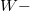﻿

New$W-$weighted concepts for continuous random variables with applications

Abstract

New concepts on fractional probability theory are introduced and some inequalities for the fractional$w-$weighted expectation and the fractional$w-$weighted variance of continuous random variables are obtained. Other fractional results related to the two orders-fractional$w-$% weighted moment are also established. Some recent results on integral inequality theory can be deduced as some special cases. At the end, some applications on the uniform random variable are given.

DOI Code: 10.1285/i15900932v37n1p23

Keywords: Integral inequalities; Riemann-Liouville integral; random variable; fractional fractional \$w-\$weighted expectation; fractional \$w-\$weighted variance; fractional \$w-\$weighed moment

Full Text: PDF